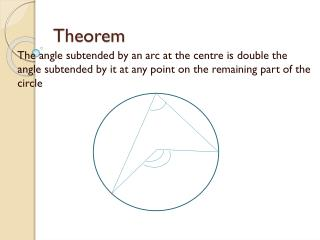DownloadDownload PresentationTheorem

# Theorem

Télécharger la présentation## Theorem

- - - - - - - - - - - - - - - - - - - - - - - - - - - E N D - - - - - - - - - - - - - - - - - - - - - - - - - - -
##### Presentation Transcript

1. Theorem The angle subtended by an arc at the centre is double the angle subtended by it at any point on the remaining part of the circle

2. The three cases We have to consider three cases • When arc PQ is minor arc • When arc PQ is a semicircle • When arc PQ is major arc

3. Case-1 Arc PQ is minor arc A O B Q P

4. Case-2 • Arc PQ is Semicircle A O Q P B

5. Case-3 • Arc PQ is major arc A P Q O B

6. Proof Given :Arc PQ of a circle subtending ∟POQ at the centre o and ∟PAQ at a point A on the remaining part of the circle. To prove: ∟POQ = 2 ∟PAQ • Construction: join AO and extend it to a point B • Proof : In all the cases ∟BOQ = ∟OAQ +∟AQO(exterior angle is equal to the sum of the interior opposite angles)

7. Angles in a segment • Angles in the same segment of the circle are equal(Theorem 10.9)

8. Theorem 10.10 • Aline segment joining two points subtends equal angles at two other points lying on the same side of the line containing the line segment, the four points lie on a circle